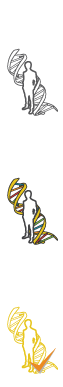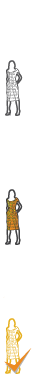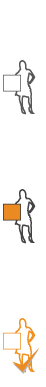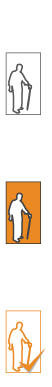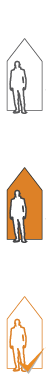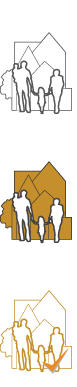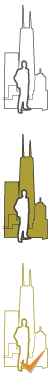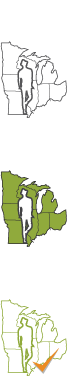#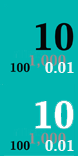Metrics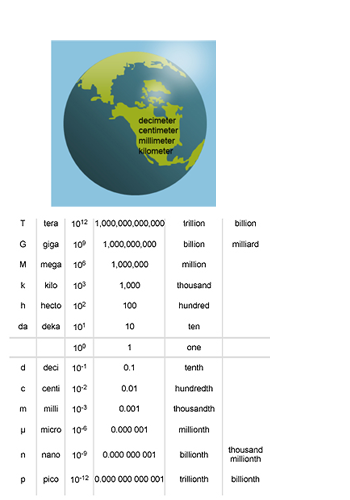Think in Tens!
Can you count to 10? We have 10 fingers…and 10 toes. The metric system is based on the powers of 10. If you can count to 10, you can know the metric system of measurement. 95% of the world’s population uses the metric system and so can you! Gabriel Mouton, from Lyons, France, is considered the “founding father” of the metric system. First proposed in 1670, the Metric System is derived from the natural systems of our earth. A portion of the Earth’s circumference, or “one ten-millionth the distance from the equator to the North Pole, originally determined the unit of length, a meter. Currently, the length of one meter is defined as 1⁄299,792,458th of a light second. The metric system uses powers of ten prefixes that are comparable to the decimal system of numbers. The most common prefixes for values above the base unit are hecto- (hundred), kilo- (thousand) and mega- (million); and the most commonly used for parts below the base value are deci- (tenth), centi- (hundredth) and milli- (thousandth).

Simply shifting the decimal point performs calculations for the metric system. Just like scientists around the world, you will find the metric system easy to use and apply! Think in tens!

Ten and powers of ten have never been so important!

## Activity 1 – Metric Measure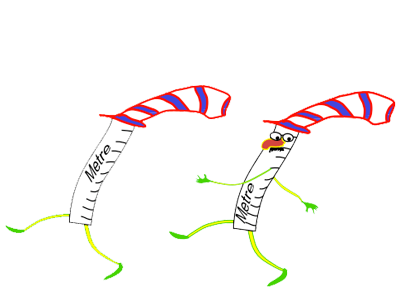Think in tens! Measure in the Metric system. Remember the activities you did in the Measure Journey? Take a second look at your room. This time estimate the length of your room in meters. Using a meter stick or metric tape measure, record the length and width of your room. Look at how long a meter is and think about how many of them (and portions of a meter) stretch across your room. Record your estimate and your answer. Repeat your experiment in other rooms and compare your findings to your answers in the Measure Journey. Were you able to improve your accuracy? Next, try and estimate other metric units: check the length of the table in front of you. Which unit should you use - meter, centimeter or millimeter? Which will do the best job? Measure with a ruler to see how close you came to your guess. Thoughtful practice will improve your understanding of the Metric system. You will find that your guesses will be more accurate once you begin to think in Metric!

## Activity 2 – Metric Modules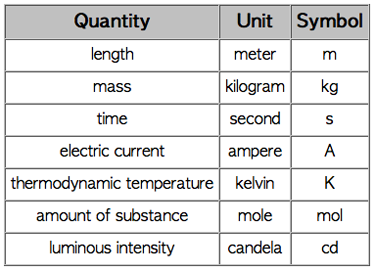Metrics, as a system of measurement, applies to quantities of just about everything we use. Metric and English systems for properties such as mass, length, volume, and temperature differ. Internationally, the metric system is the standard, but the United States still uses the English system. Converting to metric is easy once you know the basic terms, and principles:

Basic units of Metric Measurement
meter - distance
kilogram - mass
second - time
ampere - electric current
kelvin - temperature
mole - amount of substance
candela - intensity of light
Make flash cards and match units of mass, distance, time, current and temperature between the United System and the Metric System. Memorize metric units of measurement!

## Activity 3 – Metric volumes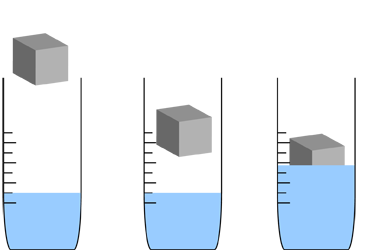Measurements of capacity or volume (liter) and mass or weight (gram) are derived from the relationship of water to the meter: 1 milliliter of water = 1 gram = 1 centimeter. Find an old plastic bottle and fill it about half full with water. Then, carefully pour about 50 ml (milliliter) of water in at a time, making a measurement mark on the bottle each time. Each mark then represents 50 ml. Next, fill the bottle with water until it is right on one of your marks. Then, take a block of clay and form it into a cube that is 5 cm (centimeter) square on all sides.Now, place your cube in the bottle of water, pay attention to how much the water rises. Does it rise 125 ml like it is supposed to? (Remember that your cube is 5 cm x 5 cm x 5 cm cubed, hence 125 cm3) If it doesn’t rise 125 ml, ask yourself why. Is your cube exactly 5 x 5 x 5? Maybe your 50 ml marks are not exactly right. Compare your answers with your friends and try your measuring skills again. Try another size, 4 cm x 4 cm x 6 cm. How many ml will make the water rise? Next, collect some coins from around the house. Measure how much area they take up by placing them in your measuring bottle.

## Activity 4 – International MetricsScientists across the globe are using the metric system to measure our world! Gabriel Mouton, from Lyons, France, is considered the founding father of the metric system. First proposed in 1670, units of the Metric System are derived from the natural systems of our earth. The unit of length is determined by a portion of the Earth’s circumference. Measures for capacity (volume) and mass are derived from that original unit of length. Each of the basic units of the Metric system relate to each other and to nature: 1 ml. (milliliter) has the same volume as 1 cubic centimeter, 1 ml. of water has a mass of about one gram and 1 L (liter) of water has a mass of about 1 kg. Larger and smaller multiples of each unit are created by multiplying or dividing the basic units by 10 and its powers. Calculations can be performed simply by shifting the decimal point. Just like scientists around the world, you will find that the Metric system is easy to use and apply!

Metric units of measure are commonly referred to as the International System of Units or SI. A series of base units define each measurement in an absolute way without directly referring to any other unit.

## Activity 5 – English to Metric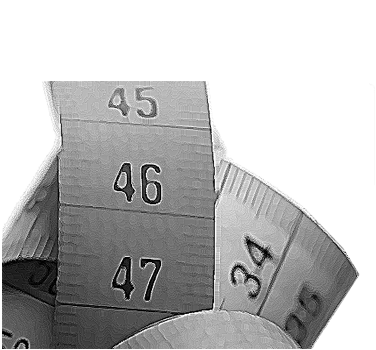Create a window to measure the World! Build a metric conversion card. First, take three pieces of heavy construction paper about 7 in by 10 in. Cut one of the sheets a little longer than it is wide, cut the other two the same size slightly wider and a little shorter than the first. Make four columns on the first piece of paper, one each for length, volume, weight and temperature. Use this metric conversion table as a guide. Copy the English-to-Metric version on one side and the Metric-to-English version on the other side. While you are copying the table, do you notice any patterns forming? The relationships between English and Metric measurements are always the same.

Next, cut four windows, the size of one conversion set, into each of the two wider pieces of paper; then, glue or tape them together to make a cover for your chart. Finally, slide your table chart in between the cover and decorate the outside. Design a colorful cover. Be sure to label each window with the correct abbreviation. Check the Metric Patrol link to be certain. Find an English measurement around your home to convert into Metric!

## Review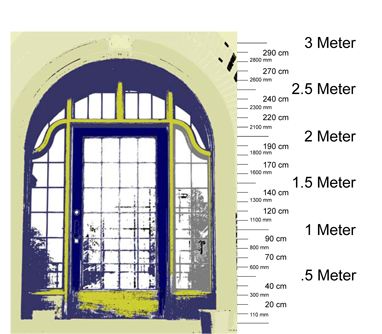• Which metric measure would you use to measure the thickness of a flower petal?
• Which is larger, a millimeter or a centimeter?
• How many feet equal a meter?
• What metric measure is our equivalent of "gallon"?
• Which is longer, a kilometer or a mile?
check answers